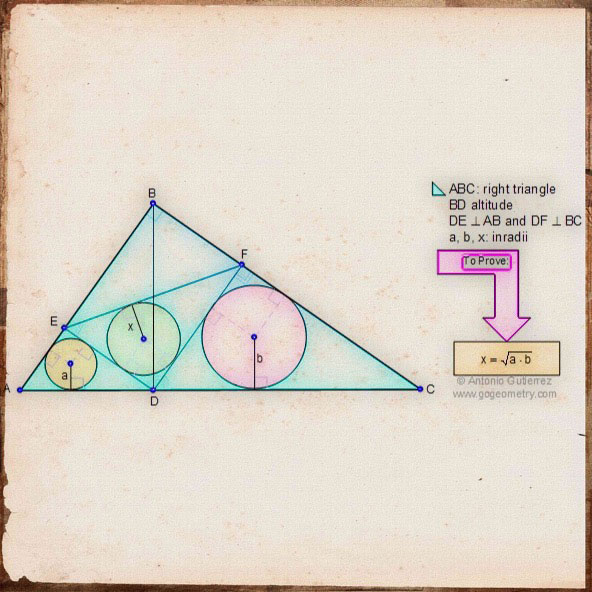# Online Geometry Problem 20: Right triangle, Altitude, Perpendiculars, Inradii, Similarity, Geometric Mean. Sketch, Software.

 In triangle ABC, angle B is a right angle. BD is the altitude, DE is perpendicular to AB and DF is perpendicular to BC. a, b, and x are the inradii of the triangles AED, DFC and EDF respectively. Prove that x is the geometric mean of a and b that is: $$x = \sqrt{a \cdot b}$$.## Sketch of problem 20 using iPad Apps.# Art of Geometry Problem 20 using Mobile Apps.

Geometric art is a form of art based on the use and application of geometric figures. A geometric figure is any set or combination of points, lines, surfaces and solids. A mobile app or mobile application software is a computer program designed to run on smartphones and tablet computers. See also the kaleidoscope of problem 20.